# Tension in a string being pulled from both ends

• AAAA
In summary, the total tension in a string being pulled on each end by 60N is 60N. This is because the forces have both magnitude and direction, and cannot simply be added together like numbers. In order to determine tension, it is best to draw a free body diagram and analyze the forces acting on the object. In the case of a string being pulled on both ends with equal force, the net force is zero and therefore the tension is the same as the force being applied on each end. If one end of the string is tied to a fixed object, the tension will be the same as the force being applied on the other end. However, if the forces applied on each end are different, the tension will also be different

## Homework Statement

If you have a string being pulled on each end by 60N, what is the total tension in the string?

N/A[/B]

## The Attempt at a Solution

The answer in my textbook says 60N, but it doesn't make a whole lot of sense to me (even though it's obviously right). If you're pulling both ends with 60 Newtons of force, why isn't it 120N of force, total, in the string? Why is it that the tension is only 60N? If one end were tied to a fixed object, and the string was pulled by 60N, then the total tension would also be 60, no? So then do you get the same result from pulling the other end rather than keeping it stationary?

If one end were to pulled by 70N, and the other by 60N, would the total tension be 70?

I find tension fairly confusing, and any help would very much appreciated.

If you tied one end of the string to the wall, and pulled the other end with a force of 60 N, what is the tension in the string?

The case where the string is being pulled with a force of 60 N from both ends does not result in a tension of 120 N, because forces have magnitude and direction. You can't simply add the two force magnitudes here and ignore that one force is acting in the opposite direction from the other.

In order to determine tension in a string or a bar or whatever, it's best to draw a free body diagram before proceeding with the analysis.

If you tied one end, and pulled the other end with 60 N, the force should be 60 N, right?

I'm not sure how to go about drawing a FBD for this question, but here it is: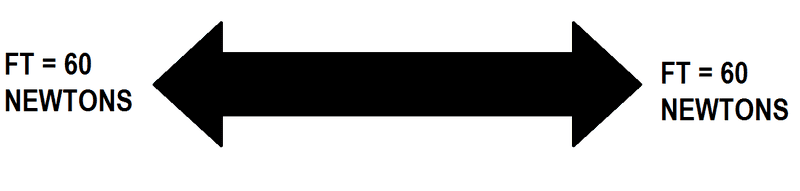If there are two forces of 60 N on each side, then isn't the net force 0? It's obviously not, but I don't see why it isn't.

What if there was 90 N of tension on one side, what is the total tension then?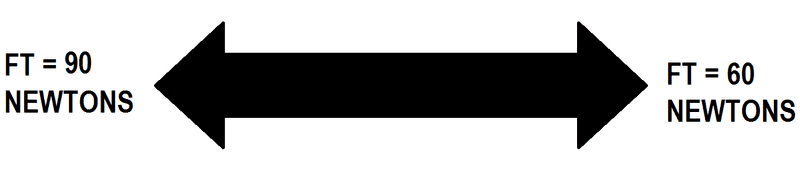AAAA said:
If one end were tied to a fixed object, and the string was pulled by 60N, then the total tension would also be 60
Suppose, you tie a weight on one end of a rope and the other end is in your hand. Then hang it from your hand. So, the rope is stationary. At some moment, you drop the rope. Then the rope falls down at an accelaration of ##9.8 m/s^2##. When, the other end of the rope was in your hand, do you think you were not exerting any force on the rope?

arpon said:
Suppose, you tie a weight on one end of a rope and the other end is in your hand. Then hang it from your hand. So, the rope is stationary. At some moment, you drop the rope. Then the rope falls down at an accelaration of 9.8m/s29.8 m/s^2. When, the other end of the rope was in your hand, do you think you were not exerting any force on the rope?

Right, so since the rope is stationary, I'm exerting a force upwards, which is equal to the acceleration by gravity, times the mass of the weight. (mass of rope isn't included because in class we've always assumed that ropes are inelastic and mass-less). So in this case, the force of tension in the rope is that value obtained by mg (or ma), despite there being two opposite forces acting as tension on the rope.

Is that what you're getting at?

Thank you both for your help so far.

AAAA said:
Is that what you're getting at?
Exactly !
AAAA said:
What if there was 90 N of tension on one side, what is the total tension then?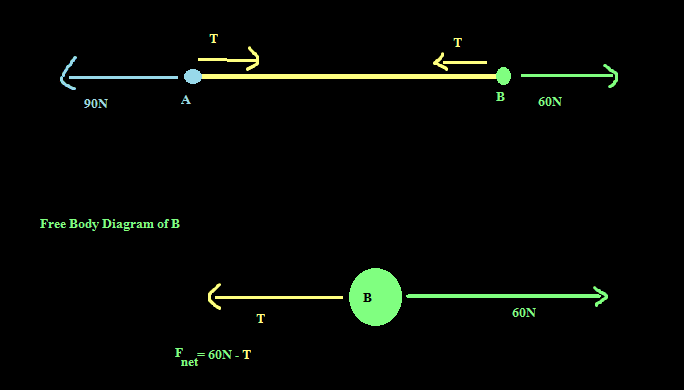And the net force on B is equal to the mass of B times the accelaration of B. And look, the force exerted on both A and B by the rope is same , i.e., T which is the tension of the rope. According to Hooke's Law, the tension of the rope is proportional to the extension. At some time, the rope completes its extension.And the tension is constant after that. As, the rope doesn't extends anymore, the accelaration of A and B will be same. Keeping these in mind, you may find another equation for A and find out the tension and also the accelaration of the system.

So what would the tension be in that "center" rope, between masses A and B? We would need to assign some arbitrary values for mass and acceleration, right? ↓ I believe you mentioned that here ↓:
Keeping these in mind, you may find another equation for A and find out the tension and also the accelaration of the system.

What if there were no masses, what if it were just a mass-less rope with two forces on both ends?

Last edited:
AAAA said:
If you tied one end, and pulled the other end with 60 N, the force should be 60 N, right?

I'm not sure how to go about drawing a FBD for this question, but here it is: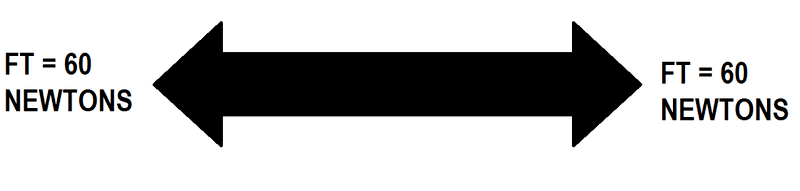If there are two forces of 60 N on each side, then isn't the net force 0? It's obviously not, but I don't see why it isn't.

Of course the net force is zero. How could it be otherwise? That's the essence of static equilibrium: there's no net force.

What if there was 90 N of tension on one side, what is the total tension then?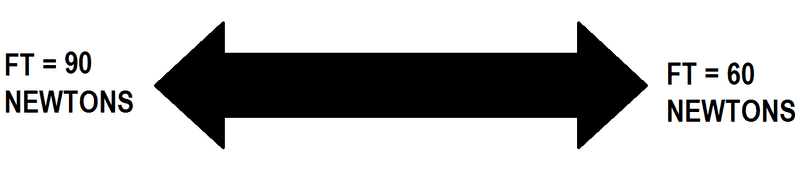This situation is not in equilibrium with only the forces shown. You can't draw a free body diagram to determine the tension here because depending on which diagram you draw, you will get inconsistent results. That's one crucial reason why free body diagrams can be used only when a body is in equilibrium.

AAAA said:
What if there were no masses, what if it were just a mass-less rope with to forces on both ends?
It is just like, you are exerting a force on a massless object. As ##F = ma##, or, ##a = \frac{F}{m}##, as ##m=0## , ##a## becomes infinity, isn't it?

SteamKing said:
Of course the net force is zero. How could it be otherwise? That's the essence of static equilibrium: there's no net force.
Okay, that makes sense.

arpon said:
It is just like, you are exerting a force on a massless object. As F=maF = ma, or, a=Fma = \frac{F}{m}, as m=0m=0 , aa becomes infinity, isn't it?
So how would I determine the force of tension in a rope with, say, 90 N of force on the left hand side and 60 N of force on the right hand side? Assuming still a mass-less, and inelastic rope.

AAAA said:
So what would the tension be in that "center" rope, between masses A and B?
Tension is same in every parts of the rope.

arpon said:
Tension is same in every parts of the rope.
Oh, okay, that makes sense.

AAAA said:
So how would I determine the force of tension in a rope with, say, 90 N of force on the left hand side and 60 N of force on the right hand side? Assuming still a mass-less, and inelastic rope.
In case of inelastic rope, the accelaration in both the ends are same.

arpon said:
In case of inelastic rope, the acceleration in both the ends are same.
Right, yeah, I remember my teacher telling us that. So all objects connected to the rope would also accelerate at the same rate.

AAAA said:
Right, yeah, I remember my teacher telling us that. So all objects connected to the rope would also accelerate at the same rate.

I was actually thinking about the accelaration. However, if you want to find the tension, think in this way, two men are exerting equal forces of 60N on the two ends of the rope in opposite direction. They are on a truck which is moving with accelaration of ##3*10^5 ms^{-2}##
So, what is the tension of the rope ? It is 60N. What is the accelaration of the rope? It's ##3*10^5 ms^{-2}## . And , suppose, the rope has a negligible mass of ##10^{-4} kg##. So, what is the net force on the rope (net force equals to mass times accelaration) ? It's ##3*10^5 ms^{-2} \cdot 10^{-4} kg = 30N##. It's almost the similar case as your question. Can you catch it?

Last edited:
AAAA said:

## Homework Statement

If you have a string being pulled on each end by 60N, what is the total tension in the string?

N/A[/B]

## The Attempt at a Solution

The answer in my textbook says 60N, but it doesn't make a whole lot of sense to me (even though it's obviously right). If you're pulling both ends with 60 Newtons of force, why isn't it 120N of force, total, in the string? Why is it that the tension is only 60N? If one end were tied to a fixed object, and the string was pulled by 60N, then the total tension would also be 60, no? So then do you get the same result from pulling the other end rather than keeping it stationary?

If one end were to pulled by 70N, and the other by 60N, would the total tension be 70?

I find tension fairly confusing, and any help would very much appreciated.

Let's consider two cases:

1) A rope is bring pulled at both ends with the same force. 60N, say.

2) A rope is attached to a wall at one end and being pulled with a force of 60N at the other.

What is the difference in these two cases? The answer is there is no difference. Newton's third law tells you that the wall must be pulling the rope with 60N.

Now, let's say that's what we mean by a tension in the rope of 60N. Both ends are being pulled with the same force.

To understand this better think of the rope as a series of links in a chain. Each pair of links is pulling the other with 60N in opposite directions.

So, you have a whole series of these pairs of 60N forces, all the way along the chain.

To take it to the next level, think about the adjacent molecules in the rope. At that level you have a large number of pairs of forces between adjacent molecules all the way along the rope.

That's what tension is and it's always a two-way thing.

Finally, to think about the non equilibrium case. If you attach one end of the rope to an object that, say, resists at 60N (friction on the floor perhaps) and pull the other at 90N, then the tension in the rope will be 90N and the object will start to accelerate.

A big conceptual difficulty is the misconception that "the tension in a string" is a force. Knowing the value of "the" tension in a string allows us make deductions about forces at both ends of it and we may consider a segment of it as a free body and make deductions about forces there. Tension has units of force, but the statement "The tension in a string is 60 Newtons" contains more information than a statement like "A force of 60 Newtons acts at point (0,0) in the upward direction". When different locations in an object have variable tension, stress, or strain then it becomes necessary to invent related definitions that apply "at a point". But the statement "the tension in a string is 60 Newtons" is a statement about a condition that is uniform at every point in the string. The value for the (uniform) tension in a string is not a force, it is information that allows us deduce the magnitude of forces at many different "points of application".

Of course when the tension in a string allows us to deduce a force, it is common to say the force "is" the tension in the string. Ordinary speech is convenient and imprecise.

•jbriggs444 and PeroK
Stephen's point becomes very important when you consider the mass of the rope and no longer assume it is massless. E.g the tension in a free hanging rope will decrease from the full weight of the rope at the top to zero at the very bottom.

PeroK said:
E.g the tension in a free hanging rope will decrease from the full weight of the rope at the top to zero at the very bottom.
What do you mean by that?

I think I'm beginning to fully understand tension (on a very high-level, of course), thank you all for your answers.

AAAA said:
What do you mean by that?

The tension in a hanging rope varies from top to bottom. Each part of the rope is holding up all the rope below it. Again, if you think about a chain rather than a rope: the force between each pair of links reduces from top to bottom.

PeroK said:
The tension in a hanging rope varies from top to bottom. Each part of the rope is holding up all the rope below it. Again, if you think about a chain rather than a rope: the force between each pair of links reduces from top to bottom.
Oh okay, that makes sense. Thanks.

I'm not sure if this warrants a completely new thread, but here goes: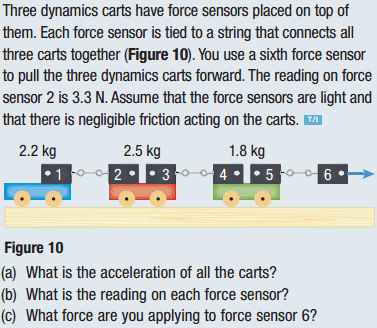I got all the answers correct for a and b.

I determined (a) from knowing that if force sensor two is having a "force" of tension pulling it back 3.3N, that is the force required to pull the very first cart (blue), so I solved for acceleration using FNET = ma, and got 1.5m/s/s.

Then, knowing acceleration of all the carts (they must accelerate at the same rate because they are all connected), and, knowing the masses of the carts, I solved for the forces on the other sensors. Also knowing that force sensors that are connected must be having the force of tension (S1/S2: 3.3N, S3/S4: 7.05N, S5/S6: 9.78N).

For c, I thought it would be 9.75N, considering that's the force detected on sensor 6, but it's not. It's ~20N, which is the sum of all forces required to pull the carts. Why is it the sum of the forces rather than the force detected by sensor 6?

AAAA said:
I'm not sure if this warrants a completely new thread, but here goes:I got all the answers correct for a and b.

I determined (a) from knowing that if force sensor two is having a "force" of tension pulling it back 3.3N, that is the force required to pull the very first cart (blue), so I solved for acceleration using FNET = ma, and got 1.5m/s/s.

Then, knowing acceleration of all the carts (they must accelerate at the same rate because they are all connected), and, knowing the masses of the carts, I solved for the forces on the other sensors. Also knowing that force sensors that are connected must be having the force of tension (S1/S2: 3.3N, S3/S4: 7.05N, S5/S6: 9.78N).

For c, I thought it would be 9.75N, considering that's the force detected on sensor 6, but it's not. It's ~20N, which is the sum of all forces required to pull the carts. Why is it the sum of the forces rather than the force detected by sensor 6?
Looks like someone is wrong, but it's not you! Your answer is correct.

Alright, thanks :D

wow, thanks for the detailed answers and questions!

I do not know the reason but I have found in one of my books that when a rope or string is pulled with equal forces on two sides ,tension in the rope is equal to either of the forces applied.Maybe this might be somewhat helpful...

I do not know the reason but I have found in one of my books that when a rope or string is pulled by equal forces on opposite sides then the tension is equal to either of the forces. Maybe this might be helpful...

Bishal said:
I do not know the reason but I have found in one of my books that when a rope or string is pulled by equal forces on opposite sides then the tension is equal to either of the forces. Maybe this might be helpful...
If it was only pulled from one end it would move and there would be no tension.

hy, i have a idea, we take the concept of equilibrium
If we hang a body of some weight with one end on string and other is fixed with something then body is in equilibrium because net force is zero due to tension and weight because by Newton third law tension in the string and weight are equal but opposite
so tension is that force which applied by fixed point so tension is a force which are applied through string on any body
so if we pull both end by 60 N then tension is string is also 60 N but total force is zero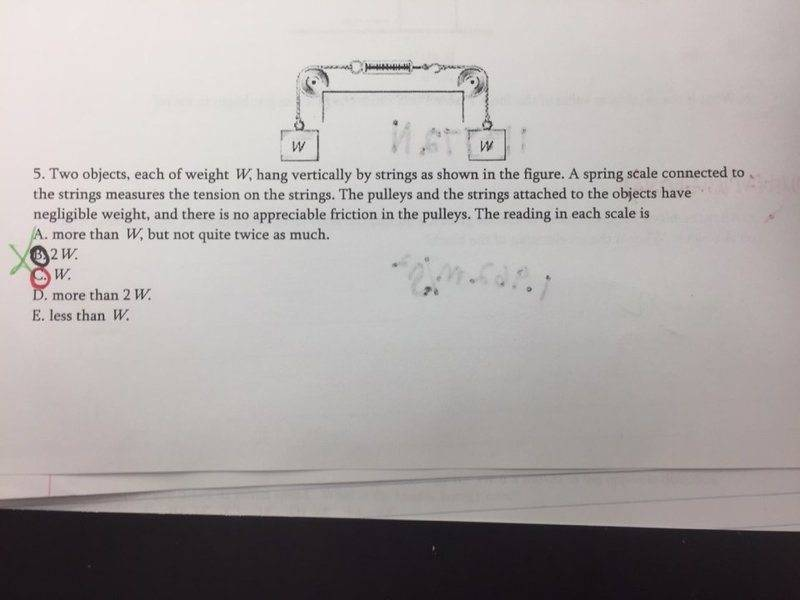I understand that the tension is equal to W, but, I do not understand why it equals W as oppose to it equaling 2W. I need to have the explanation of why tension would equal W to get points for corrections on my test.

Barrett Long said:
View attachment 109644

I understand that the tension is equal to W, but, I do not understand why it equals W as oppose to it equaling 2W. I need to have the explanation of why tension would equal W to get points for corrections on my test.

If it was ##2W## each mass would have an unbalanced upward force of ##2W - 1W## acting on it and each would acclerate upwards!